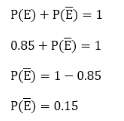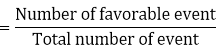or# RD Sharma Class 10 and Chapter Probability Maths Solutions

## Maths Class 10 and Chapter Probability RD Sharma SolutionsFilter
Filters :
CLASSES
•
•
•
•
•
•
•
CHAPTERS
•
• 6 More

### RD SHARMA Class 10 | PROBABILITY

In the given figure, A, B, C and D are centres of four circles that have a radius of 1 unit. If a point is selected at random from interior of square ABCD, then what is the probability that it lies in the shaded region### RD SHARMA Class 10 | PROBABILITY

One card is drawn from a pack of 52 cards, each of the 52 cards being equally likely to be drawn. Find the probability that the card drawn is: an ace(ii) redÂ  (iii) either red or king (iv) red and a king.### RD SHARMA Class 10 | PROBABILITY

The king, queen and jack of clubs are removed from a deck of 52 playing cards and the well shuffled. One card is selected from the remaining cards. Find the probability of getting. i)a heart ii)a king iii)a club iv) the 10 of hearts### RD SHARMA Class 10 | PROBABILITY

From a pack of 52 playing cards Jacks, queens, kings and aces of red colour are removed. From the remaining, a card is drawn at random. Find the probability that the card drawn is :  a black queen     (ii)  a red card   (iii)  a black jackIn this chapter, we will learn about Probability. ‘Probability’, means the number of times an event or an incident is likely to occur. Doubtnut has made learning much more comfortable and simpler where the students do not worry.

Students who follow NCERT books do not get in-depth knowledge of the subject matter. They only get to clear their basic concepts which lead them to a lot of problems while appearing in the exams. So, in this case, they try to search for the different guides or reference books that help them to clear their queries. Class 10 Maths RD Sharma Solutions helps a lot to provide the best information to the students on how to attempt the difficult questions with the best approach. So, this helps students to get the proper and clear idea of how to come up with accurate solutions.

Students who wish to score high marks in the examination should look forward to the online study materials by Doubtnut. The experienced teachers have designed the video tutorials in such a way so that the students do not have to find any problem while dealing with a particular question. Also, online study materials can be accessed by the students free of cost. So, this saves quite a lot of money and time opting for it. Students can get all clear information on how to solve even the trickiest question without any problem at all.

## Topics and subtopics of RD Sharma class 10 Chapter 13 Probability

Exercise 1: There is a probability that it will rain today is 0.85. Can you calculate the probability that it will not rain today?

Solution:

Given: Probability that it will rain P(E) = 0.85

To Find: Probability that it will not rain P(E)

Calculation: We know that sum of the probability of occurrence of an event and probability of non occurrence of an event is 1.Hence the probability that it will not rain is = 0.15

Exercise 2:  A die is thrown. Find the probability of getting:

(i) a prime number

(ii) 2 or 4

(iii) a multiple of 2 or 3

(iv) an even prime number

(v) a number greater than 5

(vi) a number lying between 2 and 6

Solution:

Given: A dice is thrown once

To Find

(i) Probability of getting a prime number

(ii) Probability of getting 2 or 4

(iii) Probability of getting a multiple of 2 or 3.

(iv) Probability of getting an even number

(v) Probability of getting a number greater than five.

(vi) Probability of lying between 2 and 6

Total number on a dice is 6.

(i) Prime number on a dice are 2, 3, 5 Total number of prime numbers on dice is 3

We know that ProbabilityHence probability of getting a prime number = 3/6 = 1/2

(ii) For getting 2 and 4 favorable outcome are 2

We know that ProbabilityHence probability of getting 2 or 4 = 2/6 = 1/3

(iii) Multiple of 2 are 3 are 2, 3, 4 and 6

Hence favorable outcome is 4

We know that ProbabilityHence probability of getting an multiple of 2 or 3 = 4/6 = 2/3

(iv) An even prime number is 2

Hence favorable outcome is 1

We know that ProbabilityHence probability of getting an even prime number 1/6

(v) A number greater than 5 is 6

Hence favorable outcome is 1

We know that ProbabilityHence probability of getting a number greater than 5 = 1/6

(vi) Total number on a dice is 6.

Number tying between 2 and 6 are 3, 4 and 5

Total number of numbers lying between 2 and 6 is 3

We know that ProbabilityHence probability of getting a number lying between 2 and 6 = 3/6 =1/2

Exercise 3:  Three coins are tossed together. Find the probability of getting:

(iii) at least one head and one tail

(iv) no tails

Solution:

Given: Three coins are tossed simultaneously.

To Find: We have to find the following probability

When three coins are tossed then the outcome will be

TTT, THT, TTH, THH. HTT, HHT, HTH, HHH.

Hence total number of outcome is 8.

(i) For exactly two head we get favorable outcome as THH, HHT ,HTH

Hence total number of favorable outcome i.e. exactly two head 3

We know that ProbabilityHence probability of getting exactly two head is 3/8

(ii) In case, at least two head we have favorable outcome as HHT, HTH, HHH ,THH

Hence total number of favorable outcome i.e. at least two head is 4

We know that ProbabilityHence probability of getting at least two head when three coins are tossed simultaneously is equal to 4/8 = 1/2

(iii) At least one head and one tail we get in case THT, TTH, THH. HTT, HHT, HTH,

Hence total number of favorable outcome i.e. at least one tail and one head is 6

We know that ProbabilityHence probability of getting at least one head and one tail is equal to 6/8 = 3/4

(iv) No tail i.e HHH

Hence total number of favorable outcome is 1

We know that ProbabilityHence probability of getting no tails is 1/8

## Importance of Doubtnut for the Students

Students can benefit a lot by opting for the perfect study materials provided by Doubtnut. This can really help them to get all the important idea on how to attempt the different problems without any worry. So, let us have a look at the different benefits that students can enjoy by opting for the best and useful study materials.

• Lots of exercises and examples included: Doubtnut has included lots of examples and exercises which helps students to get the best idea on how to come up with the perfect solution. This helps to provide all the right information on how to approach each question so that it does not take much time to come up with the right answers. So, the video tutorials really help to increase the confidence of the students where they never need to get tense at all. The regular practice of the different exercises also makes them perfect and fit for the examinations where they can pass the exams with flying colours.
• Proves to be flexible: Students can download the study materials online in pdf format. So, in this case, they can access it from anywhere no matter where they are. So, by making your perfect decision to opt for the online video tutorials can help to excel in exams no matter how difficult the question is.  This truly helps them to get rid of their fears from this subject. In other words, they develop a liking for this subject which proves to be quite useful to them.
• Provides detailed illustrations:  It is equally possible to get a detailed example of the different concepts that help to provide accurate information on how to solve different questions.  So, students do not have to worry at all once they try to look forward to practicing the questions on a regular basis.